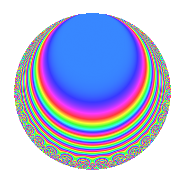# Properties

 Label 672.2.k.bLevel 672 Weight 2 Character orbit 672.k Analytic conductor 5.366 Analytic rank 0 Dimension 8 CM disc. -84 Inner twists 8

# Related objects

## Newspace parameters

 Level: $$N$$ = $$672 = 2^{5} \cdot 3 \cdot 7$$ Weight: $$k$$ = $$2$$ Character orbit: $$[\chi]$$ = 672.k (of order $$2$$ and degree $$1$$)

## Newform invariants

 Self dual: No Analytic conductor: $$5.36594701583$$ Analytic rank: $$0$$ Dimension: $$8$$ Coefficient field: 8.0.49787136.1 Coefficient ring: $$\Z[a_1, \ldots, a_{7}]$$ Coefficient ring index: $$2^{12}$$ Sato-Tate group: $\mathrm{U}(1)[D_{2}]$

## $q$-expansion

Coefficients of the $$q$$-expansion are expressed in terms of a basis $$1,\beta_1,\ldots,\beta_{7}$$ for the coefficient ring described below. We also show the integral $$q$$-expansion of the trace form.

 $$f(q)$$ $$=$$ $$q -\beta_{1} q^{3} + \beta_{5} q^{5} -\beta_{2} q^{7} -3 q^{9} +O(q^{10})$$ $$q -\beta_{1} q^{3} + \beta_{5} q^{5} -\beta_{2} q^{7} -3 q^{9} + \beta_{3} q^{11} + ( \beta_{3} + \beta_{6} ) q^{15} -\beta_{7} q^{17} + 2 \beta_{2} q^{19} + \beta_{4} q^{21} + ( \beta_{3} - \beta_{6} ) q^{23} + ( 5 - 2 \beta_{4} ) q^{25} + 3 \beta_{1} q^{27} + 2 \beta_{1} q^{31} + ( -2 \beta_{5} + \beta_{7} ) q^{33} + ( \beta_{3} + 2 \beta_{6} ) q^{35} + 2 \beta_{4} q^{37} + ( 2 \beta_{5} + \beta_{7} ) q^{41} -3 \beta_{5} q^{45} -7 q^{49} + ( \beta_{3} - 2 \beta_{6} ) q^{51} + ( -6 \beta_{1} - 2 \beta_{2} ) q^{55} -2 \beta_{4} q^{57} + 3 \beta_{2} q^{63} + ( -\beta_{5} + 2 \beta_{7} ) q^{69} + ( \beta_{3} + 3 \beta_{6} ) q^{71} + ( -5 \beta_{1} - 6 \beta_{2} ) q^{75} + ( -\beta_{5} - 2 \beta_{7} ) q^{77} + 9 q^{81} + ( -2 + 2 \beta_{4} ) q^{85} + ( 4 \beta_{5} - \beta_{7} ) q^{89} + 6 q^{93} + ( -2 \beta_{3} - 4 \beta_{6} ) q^{95} -3 \beta_{3} q^{99} +O(q^{100})$$ $$\operatorname{Tr}(f)(q)$$ $$=$$ $$8q - 24q^{9} + O(q^{10})$$ $$8q - 24q^{9} + 40q^{25} - 56q^{49} + 72q^{81} - 16q^{85} + 48q^{93} + O(q^{100})$$

Basis of coefficient ring in terms of a root $$\nu$$ of $$x^{8} + 3 x^{6} + 5 x^{4} + 12 x^{2} + 16$$:

 $$\beta_{0}$$ $$=$$ $$1$$ $$\beta_{1}$$ $$=$$ $$($$$$3 \nu^{6} + 5 \nu^{4} + 15 \nu^{2} + 26$$$$)/10$$ $$\beta_{2}$$ $$=$$ $$($$$$-2 \nu^{6} - 9$$$$)/5$$ $$\beta_{3}$$ $$=$$ $$($$$$\nu^{7} + 3 \nu^{5} + 5 \nu^{3} + 20 \nu$$$$)/4$$ $$\beta_{4}$$ $$=$$ $$($$$$\nu^{6} + 3 \nu^{4} + \nu^{2} + 6$$$$)/2$$ $$\beta_{5}$$ $$=$$ $$($$$$\nu^{7} + 3 \nu^{5} + 5 \nu^{3} + 4 \nu$$$$)/4$$ $$\beta_{6}$$ $$=$$ $$($$$$-3 \nu^{7} - 5 \nu^{5} + 5 \nu^{3} - 16 \nu$$$$)/10$$ $$\beta_{7}$$ $$=$$ $$($$$$-3 \nu^{7} - \nu^{5} - 7 \nu^{3} - 20 \nu$$$$)/4$$
 $$1$$ $$=$$ $$\beta_0$$ $$\nu$$ $$=$$ $$($$$$-\beta_{5} + \beta_{3}$$$$)/4$$ $$\nu^{2}$$ $$=$$ $$($$$$-\beta_{4} + \beta_{2} + 3 \beta_{1} - 3$$$$)/4$$ $$\nu^{3}$$ $$=$$ $$($$$$-\beta_{7} + 5 \beta_{6} + 3 \beta_{5}$$$$)/8$$ $$\nu^{4}$$ $$=$$ $$($$$$3 \beta_{4} + 3 \beta_{2} - \beta_{1} - 1$$$$)/4$$ $$\nu^{5}$$ $$=$$ $$($$$$5 \beta_{7} - 5 \beta_{6} + 7 \beta_{5} + 2 \beta_{3}$$$$)/8$$ $$\nu^{6}$$ $$=$$ $$($$$$-5 \beta_{2} - 9$$$$)/2$$ $$\nu^{7}$$ $$=$$ $$($$$$-5 \beta_{7} - 5 \beta_{6} + 2 \beta_{5} - 7 \beta_{3}$$$$)/4$$

## Character Values

We give the values of $$\chi$$ on generators for $$\left(\mathbb{Z}/672\mathbb{Z}\right)^\times$$.

 $$n$$ $$127$$ $$421$$ $$449$$ $$577$$ $$\chi(n)$$ $$1$$ $$1$$ $$-1$$ $$-1$$

## Embeddings

For each embedding $$\iota_m$$ of the coefficient field, the values $$\iota_m(a_n)$$ are shown below.

For more information on an embedded modular form you can click on its label.

Label $$\iota_m(\nu)$$ $$a_{2}$$ $$a_{3}$$ $$a_{4}$$ $$a_{5}$$ $$a_{6}$$ $$a_{7}$$ $$a_{8}$$ $$a_{9}$$ $$a_{10}$$
545.1
 1.09445 + 0.895644i 0.228425 + 1.39564i −0.228425 − 1.39564i −1.09445 − 0.895644i 1.09445 − 0.895644i 0.228425 − 1.39564i −0.228425 + 1.39564i −1.09445 + 0.895644i
0 1.73205i 0 −4.37780 0 2.64575i 0 −3.00000 0
545.2 0 1.73205i 0 −0.913701 0 2.64575i 0 −3.00000 0
545.3 0 1.73205i 0 0.913701 0 2.64575i 0 −3.00000 0
545.4 0 1.73205i 0 4.37780 0 2.64575i 0 −3.00000 0
545.5 0 1.73205i 0 −4.37780 0 2.64575i 0 −3.00000 0
545.6 0 1.73205i 0 −0.913701 0 2.64575i 0 −3.00000 0
545.7 0 1.73205i 0 0.913701 0 2.64575i 0 −3.00000 0
545.8 0 1.73205i 0 4.37780 0 2.64575i 0 −3.00000 0
 $$n$$: e.g. 2-40 or 990-1000 Embeddings: e.g. 1-3 or 545.8 Significant digits: Format: Complex embeddings Normalized embeddings Satake parameters Satake angles

## Inner twists

Char. orbit Parity Mult. Self Twist Proved
1.a Even 1 trivial yes
84.h Odd 1 CM by $$\Q(\sqrt{-21})$$ yes
3.b Odd 1 yes
4.b Odd 1 yes
7.b Odd 1 yes
12.b Even 1 yes
21.c Even 1 yes
28.d Even 1 yes

## Hecke kernels

This newform can be constructed as the intersection of the kernels of the following linear operators acting on $$S_{2}^{\mathrm{new}}(672, [\chi])$$:

 $$T_{5}^{4} - 20 T_{5}^{2} + 16$$ $$T_{43}$$